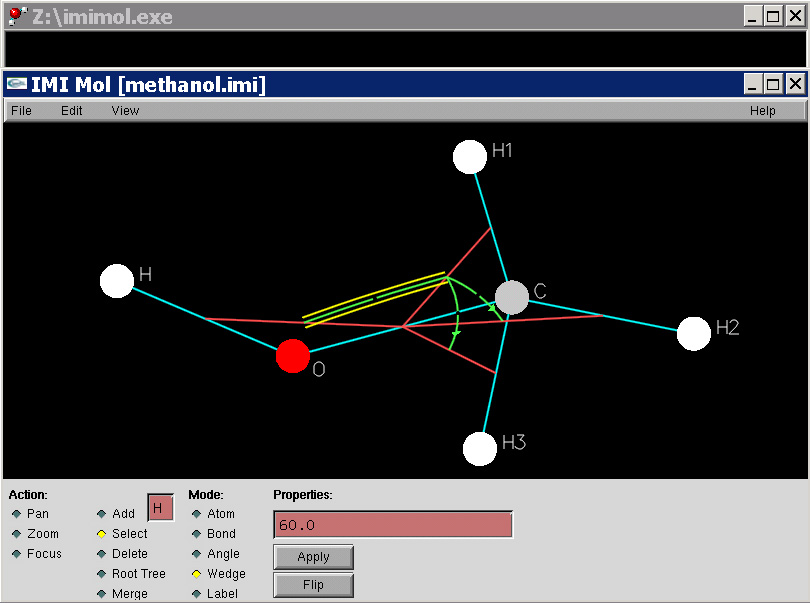Daniel B. Dix (Ph.D. in Mathematics, University of Chicago, 1988) Analysis and Applied Math.

E-mail:
dix@math.sc.edu
Mail:
Daniel B. Dix
Department of Mathematics
University of South Carolina
Columbia, SC 29208
USA
Telephone:
(803) 777-7525
FAX:
(803) 777-3783

Research interests include:
• mathematical chemistry, molecular quantum mechanics, molecular geometry, molecular dynamics;
• protein dynamics and folding, statistical mechanics.
• mathematical cellular biology, systems biology.
• initial value problems for partial differential equations governing the evolution of nonlinear waves, asymptotic behavior of solutions, solutions with special symmetry, completely integrable equations, and solitons;

• Curriculum Vita
Teaching
Research
Some papers, proposals, and preprints:
IMIMOL: A Computer Program for three dimensional Z-system (i.e. molecule) building, editing, manipulation, and visualization, and for molecular geometry computations. IMIMOL allows the user to visually organize a molecular system on a two-dimensional canvas in a way which also allows its three dimensional geometry to be fully specified. Other programs such as RASMOL or VMD should be used to visualize the molecular system in three dimensions based on files exported by IMIMOL.IMIMOL was written by Scott Johnson at the Industrial Mathematics Institute, Department of Mathematics, University of South Carolina. All rights reserved.
Berry Phase in the Molecular System H3. Master's Thesis of Jialiang Wu. This thesis presents a comprehensive treatment of the mathematical foundations of the quantum mechanics of molecules, assuming the Born-Oppenheimer approximation. In particular it treats the molecular system H3, which consists of three protons and three electrons. The mathematical formalism of quantum mechanics is developed from first principles, and elementary examples of quantum systems, such as spin systems, the hydrogen atom and molecule are worked out as illustrations of the formalism. Molecular symmetry groups and their representation theory are also treated. The coordinate systems in the shape space of three nuclei are studied. Vector bundles and connections are developed and applied to the Berry-Simon connection in the complex vector bundle over the shape space whose fibres are the energy ground state eigenspaces. ``Berry phase'' is then interpreted as holonomy associated to parallel translation in the usual sense of differential geometry.
Building Geometric Models of Biological Molecules. Master's Thesis of Haruna Katayama. This thesis applies Z-system theory to a large biomolecular system, namely the Light Harvesting Complex II (LH2) in the photosynthetic bacterium Rhodobacter Sphaeroides. An elementary mathematical account of Z-system theory is given and applied first to a simple molecular system of sodium bicarbonate dissolved in water. The molecular biology and the chemistry of the light harvesting complex are discussed from first principles. Also, since there is no X-ray crystal structure of LH2 in Rhodobacter Sphaeroides, the thesis discusses the process of homology modelling whereby molecular structures can be transferred between species sharing a high degree of amino acid sequence similarity. The process of building a labelled Z-system for LH2 is described in detail and the resulting structure is evaluated in terms of the presence or absence of steric conflicts.
Stochastic Models of Gene Expression. Master's Thesis of Lanjia Lin. This thesis presents a detailed account of the mathematical theory of the stochastic processes which are used to study gene expression in cells. The molecular biology of transcription of genes and translation of mRNAs into proteins is reviewed. A bipartite graph formalism, called a labeled Petri Net, is used to describe any system of interreacting chemicals. This can be used to define the corresponding State Graph, a directed graph with no loops and whose edges are labeled with positive numbers. A continuous time Markov process is defined which amounts to a random walk on the State Graph, where the times between transitions are also random. The time dependent probability distribution of this process satisfies a system of ordinary differential equations called the Master Equations. The theory of the Master equations is studied at some depth. A particular model of a self-regulating gene circuit is formulated and some simple simulations are performed using Jarnac.
Classifying Three-Fold Symmetric Hexagons. Master's Thesis of Amanda Gantt. This thesis studies the shapes that a hexagon (not necessarily planar) can assume in three dimensional space if all six side lengths and all six angles are specified. Actually this thesis only does this if the six lenths are l0, l1, l0, l1, l0, l1 as one cycles around the hexagon, and the six angles are theta0, theta1, theta0, theta1, theta0, theta1 as one cycles around the hexagon. This is what is meant by a three-fold symmetric hexagon. Polynomial equations are derived for the most general possible solution and explicit families of solutions are found. One family forms a trefoil knot which has an axis of three-fold rotational symmetry. The equations are derived and solved using Maple worksheets, which accompany the thesis, and allow the reader to view each type of hexagon in a rotatable three dimensional form.
Graduate Students Wanted! Some proposed research projects are as follows.
• Mass flux equations associated to a Petri Net and the steady state solutions of the associated Master equations, with applications to the control of metabolic networks in living cells.
• Ph.D. Project: Singularities in the Born-Oppenheimer potential energy function for the molecular system H3 near the Li atom limit.
• Ph.D. Project: The classical limit of the quantum dynamics of nuclei subject to an electronic potential energy surface and a gauge potential arising from geometric phase effects.
• Ph.D. Project: Coordinates systems in the shape space of flexible molecular rings, with applications to the dynamics of loop regions in proteins.
• Ph.D. Project: Large Motions in Protein Machines: Geodesics of the kinetic energy Riemannian metric on shape space within the Rammachandran set.